Next: 3.3 Electromigration Induced Stress Up: 3.2 Material Transport Equations Previous: 3.2.1 Fast Diffusivity Paths

## 3.2.2 Anisotropic Diffusivity: Diffusion Dependence on Stress

Residual mechanical stresses are introduced on interconnect lines as a result of the fabrication process flow . These stresses can be very high, significantly affecting the diffusion coefficient of the interconnect metal atoms .

Typically, the diffusion dependence on stress is taken into account through a simple modification of the usual diffusion coefficient expression (2.10) to [54,97]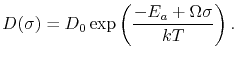(3.13)

In this way, (3.13) describes the effect of a scalar hydrostatic stress on the scalar diffusion coefficient. However, Flynn  pointed out that, for a homogeneously deformed cubic crystal with strain field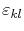, there is an additional contribution proportional toto the diffusion of the form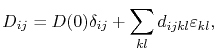(3.14)

where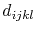is the elastodiffusion tensor, which can be experimentally determined. Here, the simple scalar diffusivity is replaced by a tensorial quantity. Consequently, in the presence of a stress field the diffusivity can now become anisotropic, depending on the properties of.

Based on microscopic lattice theory, Dederichs and Schroeder  showed that the diffusivity in a simple face-centered cubic crystal (fcc) follows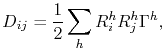(3.15)

where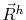is the jump vector for a hopand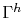is the corresponding jump rate. From classical thermodynamics the jump rate is given by [59,144]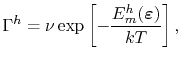(3.16)

where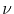is the Debye frequency (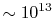s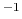for metals ) and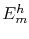is the migration energy barrier. The presence of an external strain field affects the jump rate by changing the migration energy barrier according to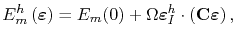(3.17)

where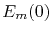is the migration energy barrier in the absence of an external field,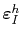is the induced strain,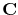is the elasticity tensor, and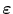is the applied external strain. For a fcc crystal the elasticity tensor has the form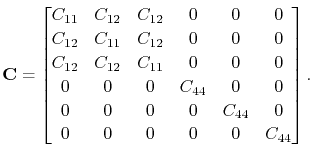(3.18)

Combining (3.16) and (3.17) one obtains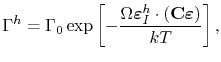(3.19)

with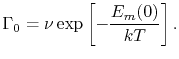(3.20)

Considering a single vacant point defect in a crystal lattice, the local volumetric strain induced by the presence of this vacancy is given by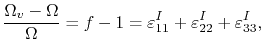(3.21)

where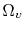is the vacancy volume and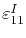,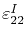, and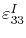are the induced strains in the given directions. Due to the symmetry of a vacant point defect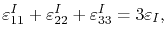(3.22)

which together with (3.21) yields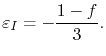(3.23)

Thus, the induced strain tensor becomes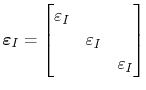(3.24)

and the induced strain vector is determined by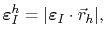(3.25)

where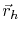is a jump unit vector for a hop.

Normalizing (3.15) with the jump distance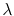and summing over the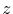nearest neighbors (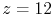for fcc crystals) one obtains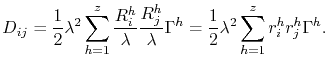(3.26)

Substituting (3.19) in this equation, the diffusivity tensor in the presence of a external stress field is determined through the expression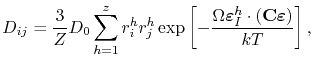(3.27)

where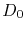is given by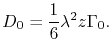(3.28)

In order to take into account the anisotropy of diffusion, the scalar diffusivity,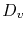, in the flux equation (3.9) is replaced by the stress dependent diffusivity tensor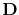yielding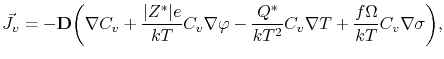(3.29)

withcalculated via (3.27). In this way, the development of mechanical stress can lead to anisotropic diffusion in the interconnect line, therefore, affecting the material transport along the line under electromigration.Next: 3.3 Electromigration Induced Stress Up: 3.2 Material Transport Equations Previous: 3.2.1 Fast Diffusivity Paths

R. L. de Orio: Electromigration Modeling and Simulation